## Blank final in Java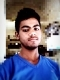Updated on 18-Jun-2020 12:40:21
In Java, a final variable can a be assigned only once. It can be assigned during declaration or at a later stage. A final variable if not assigned any value is treated as a blank final variable. Following are the rules governing initialization of a blank final variable.A blank instance level final variable cannot be left uninitialized.The blank Instance level final variable must be initialized in each constructor.The blank Instance level final variable cannot be initialized in class methods.A blank static final variable cannot be left uninitialized.The static final variable must be initialized in a static block.A static final variable cannot ... Read More

## Java program to find the area of a square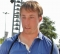Updated on 13-Mar-2020 07:33:41
A square is a rectangle whose length and breadth are same. Therefore, Area of a rectangle is the square of its length. so, to calculate the area of a squareGet the length of the square form the user.Calculate its square.Print the square.Exampleimport java.util.Scanner; public class AreaOfSquare {    public static void main(String args[]){       int length, area;       Scanner sc = new Scanner(System.in);       System.out.println("Enter the length of the square ::");       length = sc.nextInt();       area = length* length;       System.out.println("Area of the square is ::"+area);   ... Read More

## Write an example to find whether a given string is palindrome using recursion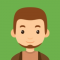Updated on 13-Mar-2020 07:31:14
Recursion is the process of repeating items in a self-similar way. In programming languages, if a program allows you to call a function inside the same function, then it is called a recursive call of the function.Following is an example to find palindrome of a given number using recursive function.Examplepublic class PalindromeRecursion {    public static boolean isPalindrome(String str){       if(str.length() == 0 ||str.length()==1){          return true;       }       if(str.charAt(0) == str.charAt(str.length()-1)){          return isPalindrome(str.substring(1, str.length()-1));       }       return false; ... Read More

## Java program to find the sum of elements of an array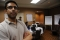Updated on 13-Mar-2020 07:32:17
To find the sum of elements of an array.create an empty variable. (sum)Initialize it with 0 in a loop.Traverse through each element (or get each element from the user) add each element to sum.Print sum.Exampleimport java.util.Arrays; import java.util.Scanner; public class SumOfElementsOfAnArray {    public static void main(String args[]){       System.out.println("Enter the required size of the array :: ");       Scanner s = new Scanner(System.in);       int size = s.nextInt();       int myArray[] = new int [size];       int sum = 0;       System.out.println("Enter the elements of the array one by one ");       for(int i=0; i

## Java program to print Fibonacci series of a given number.Updated on 13-Mar-2020 07:25:42
Recursion is the process of repeating items in a self-similar way. In programming languages, if a program allows you to call a function inside the same function, then it is called a recursive call of the function.ExampleFollowing is an example to find Fibonacci series of a given number using a recursive functionpublic class FibonacciSeriesUsingRecursion {    public static long fibonacci(long number) {       if ((number == 0) || (number == 1)) return number;          else return fibonacci(number - 1) + fibonacci(number - 2);       }       public static void main(String[] args) {          for (int counter = 0; counter

## Java program to find the factorial of a given number using recursionUpdated on 30-Jul-2019 22:30:22
Recursion is the process of repeating items in a self-similar way. In programming languages, if a program allows you to call a function inside the same function, then it is called a recursive call of the function.Following is an example to find the factorial of a given number using a recursive function.import java.util.Scanner; public class ab21_FactorialUsingRecursion { public static long factorial(int i) { if(i

## Java program to print Pascal's triangle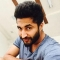Updated on 13-Mar-2020 07:24:47
Pascal's triangle is one of the classic example taught to engineering students. It has many interpretations. One of the famous one is its use with binomial equations.All values outside the triangle are considered zero (0). The first row is 0 1 0 whereas only 1 acquire a space in Pascal’s triangle, 0s are invisible. Second row is acquired by adding (0+1) and (1+0). The output is sandwiched between two zeroes. The process continues till the required level is achieved.AlgorithmTake a number of rows to be printed, n.Make outer iteration I for n times to print rows.Make inner iteration for J ... Read More

## Java program to generate and print Floyd’s triangleUpdated on 13-Mar-2020 07:22:18
Floyd's triangle, named after Robert Floyd, is a right-angled triangle, which is made using natural numbers. It starts at 1 and consecutively selects the next greater number in the sequence.AlgorithmTake a number of rows to be printed, n.Make outer iteration I for n times to print rowsMake inner iteration for J to IPrint KIncrement KPrint NEWLINE character after each inner iterationExampleimport java.util.Scanner; public class FloyidsTriangle {    public static void main(String args[]){       int n, i, j, k = 1;       System.out.println("Enter the number of lines you need in the FloyidsTriangle");       Scanner sc ... Read More

## Java program to generate random numbers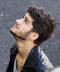Updated on 13-Mar-2020 07:20:16
The java.util.Random class instance is used to generate a stream of pseudorandom numbers.The class uses a 48-bit seed, which is modified using a linear congruential formula.The algorithms implemented by class Random use a protected utility method that on each invocation can supply up to 32 pseudo randomly generated bits.The nextInt() method of this class is used to get the next pseudorandom, uniformly distributed int value from this random number generator's sequence.Exampleimport java.util.*; public class RandomNumberGeneration {    public static void main( String args[] ) {       // create random object       Random randomno = new Random(); ... Read More

## Java program to delete duplicate characters from a given StringUpdated on 13-Mar-2020 07:18:07
The interface Set does not allow duplicate elements, therefore, create a set object and try to add each element to it using the add() method in case of repetition of elements this method returns false −If you try to add all the elements of the array to a Set, it accepts only unique elements so, to find duplicate characters in a given stringConvert it into a character array.Try to insert elements of the above-created array into a hash set using add method.If the addition is successful this method returns true.Since Set doesn't allow duplicate elements this method returns 0 when ... Read More
1 2 3 4 5 ... 15 Next